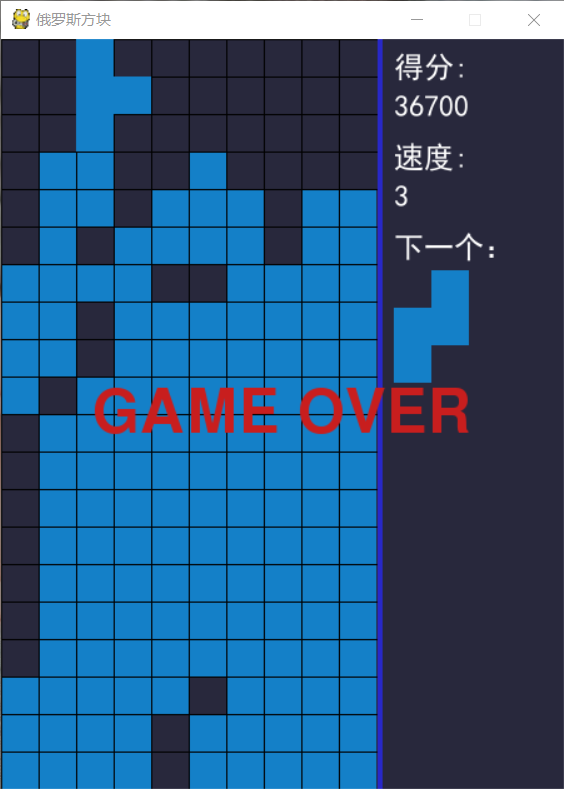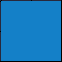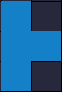# 【pygame】Python 不到 300 行代码实现俄罗斯方块## 外形

import sysimport pygamefrom pygame.locals import *SIZE = 30  # 每个小方格大小BLOCK_HEIGHT = 20  # 游戏区高度BLOCK_WIDTH = 10   # 游戏区宽度BORDER_WIDTH = 4   # 游戏区边框宽度BORDER_COLOR = (40, 40, 200)  # 游戏区边框颜色SCREEN_WIDTH = SIZE * (BLOCK_WIDTH + 5)  # 游戏屏幕的宽SCREEN_HEIGHT = SIZE * BLOCK_HEIGHT      # 游戏屏幕的高BG_COLOR = (40, 40, 60)  # 背景色BLACK = (0, 0, 0)def print_text(screen, font, x, y, text, fcolor=(255, 255, 255)):    imgText = font.render(text, True, fcolor)    screen.blit(imgText, (x, y))def main():    pygame.init()    screen = pygame.display.set_mode((SCREEN_WIDTH, SCREEN_HEIGHT))    pygame.display.set_caption('俄罗斯方块')    font1 = pygame.font.SysFont('SimHei', 24)  # 黑体24    font_pos_x = BLOCK_WIDTH * SIZE + BORDER_WIDTH + 10  # 右侧信息显示区域字体位置的X坐标    font1_height = int(font1.size('得分'))    score = 0           # 得分    while True:        for event in pygame.event.get():            if event.type == QUIT:                sys.exit()        # 填充背景色        screen.fill(BG_COLOR)        # 画游戏区域分隔线        pygame.draw.line(screen, BORDER_COLOR,                         (SIZE * BLOCK_WIDTH + BORDER_WIDTH // 2, 0),                         (SIZE * BLOCK_WIDTH + BORDER_WIDTH // 2, SCREEN_HEIGHT), BORDER_WIDTH)        # 画网格线 竖线        for x in range(BLOCK_WIDTH):            pygame.draw.line(screen, BLACK, (x * SIZE, 0), (x * SIZE, SCREEN_HEIGHT), 1)        # 画网格线 横线        for y in range(BLOCK_HEIGHT):            pygame.draw.line(screen, BLACK, (0, y * SIZE), (BLOCK_WIDTH * SIZE, y * SIZE), 1)        print_text(screen, font1, font_pos_x, 10, f'得分: ')        print_text(screen, font1, font_pos_x, 10 + font1_height + 6, f'{score}')        print_text(screen, font1, font_pos_x, 20 + (font1_height + 6) * 2, f'速度: ')        print_text(screen, font1, font_pos_x, 20 + (font1_height + 6) * 3, f'{score // 10000}')        print_text(screen, font1, font_pos_x, 30 + (font1_height + 6) * 4, f'下一个：')        pygame.display.flip()if __name__ == '__main__':    main()

## 方块I 型O 型T 型S 型Z 型L 型J 型

['.0..', '.0..', '.0..', '.0..']

['....', '....', '0000', '....']['.0..', '.0..', '.0..', '.0..']

['.0.', '000', '...']

game_area = [['.'] * BLOCK_WIDTH for _ in range(BLOCK_HEIGHT)]

cur_block = None   # 当前下落方块cur_pos_x, cur_pos_y = 0, 0  # 当前下落方块的坐标

from collections import namedtuplePoint = namedtuple('Point', 'X Y')Block = namedtuple('Block', 'template start_pos end_pos name next')# S形方块S_BLOCK = [Block(['.00',                  '00.',                  '...'], Point(0, 0), Point(2, 1), 'S', 1),           Block(['0..',                  '00.',                  '.0.'], Point(0, 0), Point(1, 2), 'S', 0)]

BLOCKS = {'O': O_BLOCK,          'I': I_BLOCK,          'Z': Z_BLOCK,          'T': T_BLOCK,          'L': L_BLOCK,          'S': S_BLOCK,          'J': J_BLOCK}def get_block():    block_name = random.choice('OIZTLSJ')    b = BLOCKS[block_name]    idx = random.randint(0, len(b) - 1)    return b[idx]# 获取旋转后的方块def get_next_block(block):    b = BLOCKS[block.name]    return b[block.next]

def _judge(pos_x, pos_y, block):    nonlocal game_area    for _i in range(block.start_pos.Y, block.end_pos.Y + 1):        if pos_y + block.end_pos.Y >= BLOCK_HEIGHT:            return False        for _j in range(block.start_pos.X, block.end_pos.X + 1):            if pos_y + _i >= 0 and block.template[_i][_j] != '.' and game_area[pos_y + _i][pos_x + _j] != '.':                return False    return True

## 停靠

def _dock():    nonlocal cur_block, next_block, game_area, cur_pos_x, cur_pos_y, game_over    for _i in range(cur_block.start_pos.Y, cur_block.end_pos.Y + 1):        for _j in range(cur_block.start_pos.X, cur_block.end_pos.X + 1):            if cur_block.template[_i][_j] != '.':                game_area[cur_pos_y + _i][cur_pos_x + _j] = '0'    if cur_pos_y + cur_block.start_pos.Y <= 0:        game_over = True    else:        # 计算消除        remove_idxs = []        for _i in range(cur_block.start_pos.Y, cur_block.end_pos.Y + 1):            if all(_x == '0' for _x in game_area[cur_pos_y + _i]):                remove_idxs.append(cur_pos_y + _i)        if remove_idxs:            # 消除            _i = _j = remove_idxs[-1]            while _i >= 0:                while _j in remove_idxs:                    _j -= 1                if _j < 0:                    game_area[_i] = ['.'] * BLOCK_WIDTH                else:                    game_area[_i] = game_area[_j]                _i -= 1                _j -= 1        cur_block = next_block        next_block = blocks.get_block()        cur_pos_x, cur_pos_y = (BLOCK_WIDTH - cur_block.end_pos.X - 1) // 2, -1 - cur_block.end_pos.Y

Python：游戏：贪吃蛇

Python：游戏：扫雷（附源码）

Python：游戏：五子棋之人机对战

------------------------------------ END ------------------------------------posted @ 2019-01-04 07:52  丹枫无迹  阅读(25427)  评论(13编辑  收藏  举报